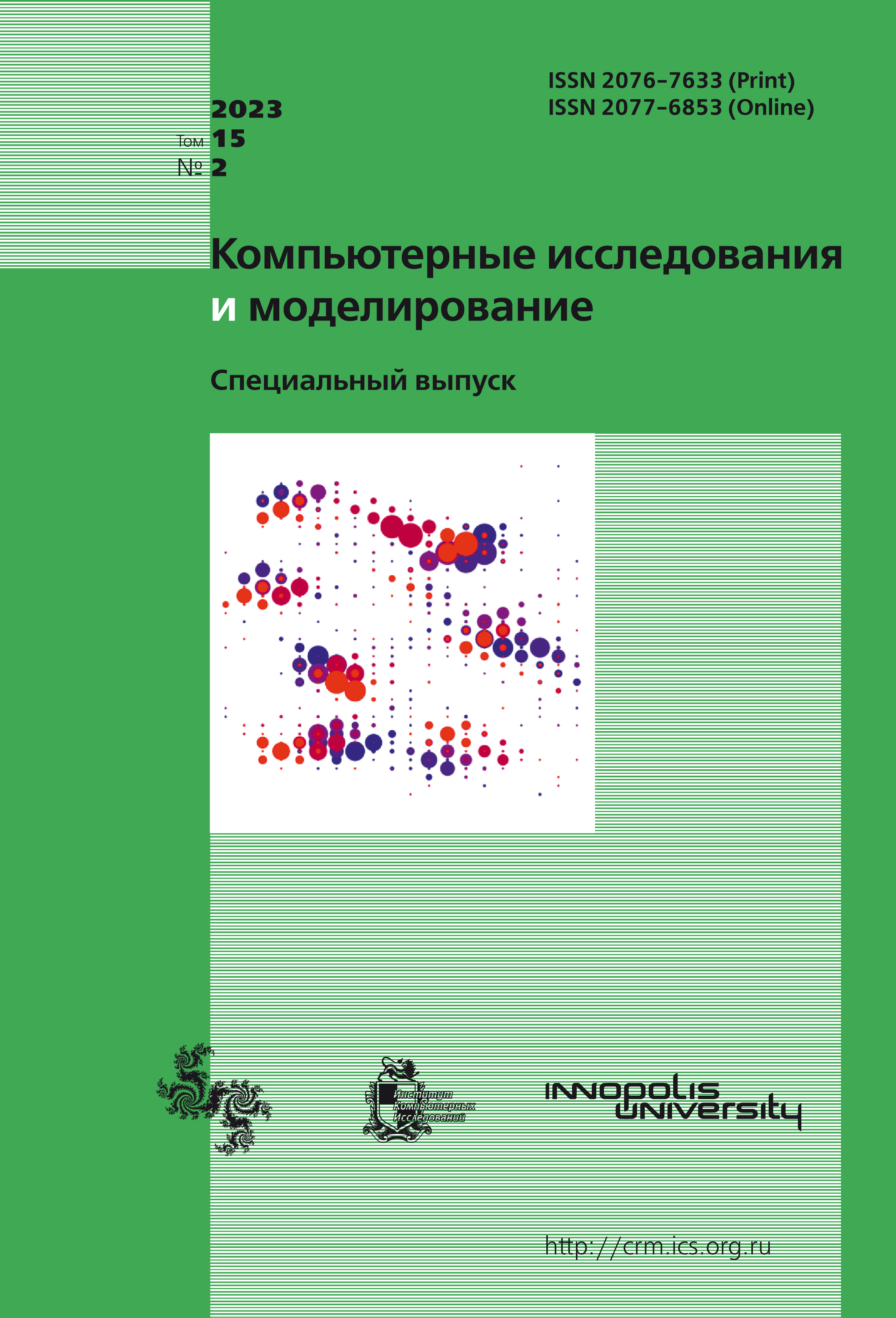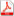Latest issue Issue 2, 2023 Vol. 15

# All issues

Survey of convex optimization of Markov decision processespdf (310K)

This article reviews both historical achievements and modern results in the field of Markov Decision Process (MDP) and convex optimization. This review is the first attempt to cover the field of reinforcement learning in Russian in the context of convex optimization. The fundamental Bellman equation and the criteria of optimality of policy — strategies based on it, which make decisions based on the known state of the environment at the moment, are considered. The main iterative algorithms of policy optimization based on the solution of the Bellman equations are also considered. An important section of this article was the consideration of an alternative to the $Q$-learning approach — the method of direct maximization of the agent’s average reward for the chosen strategy from interaction with the environment. Thus, the solution of this convex optimization problem can be represented as a linear programming problem. The paper demonstrates how the convex optimization apparatus is used to solve the problem of Reinforcement Learning (RL). In particular, it is shown how the concept of strong duality allows us to naturally modify the formulation of the RL problem, showing the equivalence between maximizing the agent’s reward and finding his optimal strategy. The paper also discusses the complexity of MDP optimization with respect to the number of state–action–reward triples obtained as a result of interaction with the environment. The optimal limits of the MDP solution complexity are presented in the case of an ergodic process with an infinite horizon, as well as in the case of a non-stationary process with a finite horizon, which can be restarted several times in a row or immediately run in parallel in several threads. The review also reviews the latest results on reducing the gap between the lower and upper estimates of the complexity of MDP optimization with average remuneration (Averaged MDP, AMDP). In conclusion, the real-valued parametrization of agent policy and a class of gradient optimization methods through maximizing the $Q$-function of value are considered. In particular, a special class of MDPs with restrictions on the value of policy (Constrained Markov Decision Process, CMDP) is presented, for which a general direct-dual approach to optimization with strong duality is proposed.

Keywords: MDP, convex optimization, $Q$-learning, linear programming, policy gradient methods
Citation in English: Rudenko V.D., Yudin N.E., Vasin A.A. Survey of convex optimization of Markov decision processes // Computer Research and Modeling, 2023, vol. 15, no. 2, pp. 329-353
Citation in English: Rudenko V.D., Yudin N.E., Vasin A.A. Survey of convex optimization of Markov decision processes // Computer Research and Modeling, 2023, vol. 15, no. 2, pp. 329-353
DOI: 10.20537/2076-7633-2023-15-2-329-353

Full-text version of the journal is also available on the web site of the scientific electronic library eLIBRARY.RU

The journal is included in the Russian Science Citation Index

The journal is included in the List of Russian peer-reviewed journals publishing the main research results of PhD and doctoral dissertations.

International Interdisciplinary Conference "Mathematics. Computing. Education"

The journal is included in the RSCI

Indexed in Scopus